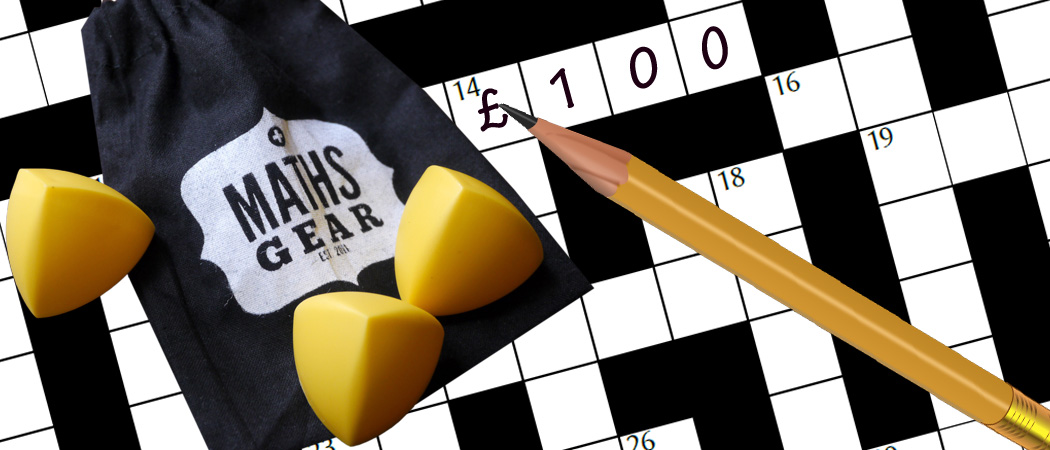# Prize crossnumber, Issue 04

Can you solve it?Our original prize crossnumber is featured on pages 48 and 49 of Issue 04.

### Rules

• Although many of the clues have multiple answers, there is only one solution to the completed crossnumber. As usual, no numbers begin with 0. Use of Python, OEIS, Wikipedia, etc. is advised for some of the clues.
• One randomly selected correct answer will win a £100 Maths Gear goody bag. Three randomly selected runners up will win a Chalkdust t-shirt. The prizes have been provided by Maths Gear, a website that sells nerdy things worldwide, with free UK shipping. Find out more at mathsgear.co.uk
• To enter, submit the sum of the across clues via this form by 7 January 2017. Only one entry per person will be accepted. Winners will be notified by email and announced on our blog by 21 January 2017.

### Crossnumber

Crossnumber #4, set by Humbug:

### Clues

#### Across

• 1. This number is a multiple of one of the two-digit answers in the crossnumber and shares no factors with the other two-digit answers. (4)
• 3. The sum of 15D, 9A and 6D. (4)
• 5. 142 less than 5D. (4)
• 8. The product of the three largest two-digit answers in this crossnumber. (5)
• 9. This number is equal to the number of digits in its factorial. (2)
• 11. A Sierpiński number.* (6)
• 12. The sum of the reciprocals of this number’s non-zero digits is one. (4)
• 13. Twice 7D. (4)
• 16. A counterexample to the conjecture that every odd number can be written in the form $p+2a^2$, where $p$ is 1 or a prime and $a$ is an integer. (4)
• 17. A second counterexample to the conjecture in 16A. (4)
• 19. A multiple of 717. (9)
• 23. Equal to another answer in this crossnumber. (9)
• 25. A palindrome. (4)
• 26. Less than 15D. (4)
• 27. 12A backwards. (4)
• 30. The sum of this number’s digits is 5. (4)
• 32. The first letter of each digit of this number is the same as the last letter of the previous digit. (6)
• 33. A number that is three times the sum of its digits. (2)
• 34. Integer part of the square root of a Fibonacci number. (5)
• 35. The sum of 9A, 27A, 27D and 28D. (4)
• 36. 1$\tfrac12$ times 29D. (4)
• 37. One less than a multiple of 30A. (4)

#### Down

• 1. A multiple of 1A. (4)
• 2. A factor of 1A. (4)
• 3. A positive integer whose square is the sum of 50 consecutive squares. (4)
• 4. All the digits of this number are the same. (9)
• 5. Half the difference between 5A and 13A. (4)
• 6. The middle two digits of 3A. (2)
• 7. 808 more than 5A. (4)
• 10. The largest number that is not the sum of two abundant numbers. (5)
• 14. The remainder when this number to the power of 91 is divided by 18,793,739 is 12A. (6)
• 15. 1D more than 26A. (4)
• 18. An anagram of 31D. (4)
• 19. The sum of this number’s digits is one less than 6D. (4)
• 20. The lowest common multiple of 7D and 5A. (6)
• 21. Equal to another answer in this crossnumber. (9)
• 22. The smallest number that appears eight times in Pascal’s triangle. (4)
• 24. The maximum number of regions that can be formed by joining 27 points on a circle with straight lines. (5)
• 27. The number of straight lines that go through at least two points of a 10$\times$10 grid of points. (4)
• 28. The sum of four consecutive positive cubes. (4)
• 29. Five less than a palindrome. (4)
• 30. The sum of 18D and 31D. (4)
• 31. An anagram of 18D. (4)
• 34. A number whose square root is equal to the sum of its digits. (2)

*A Sierpiński number is an odd number, $k$, such that for all integers $n$, $k\times2^n+1$ is not prime.

Abundant numbers are numbers which are smaller than the sum of their proper divisors.